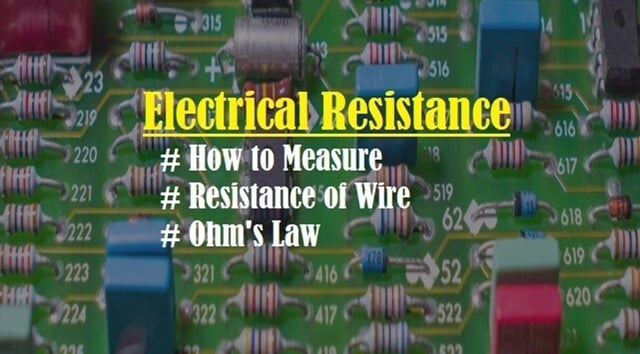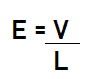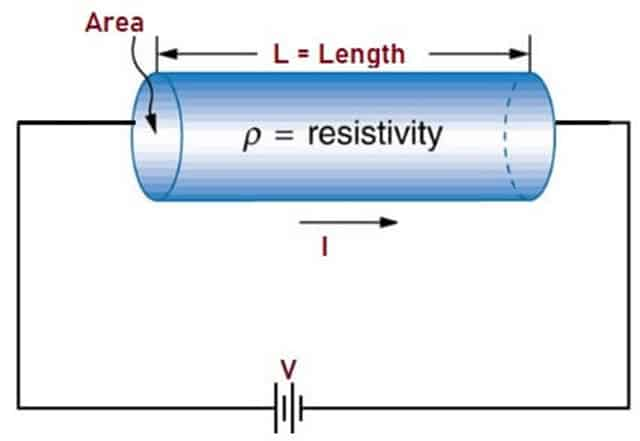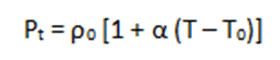Monday, September 20, 2021
HomeElectrical ResistanceElectrical Resistance - How to Measure, OHM as Function of Temperature

# Electrical Resistance – How to Measure, OHM as Function of Temperature

Electrical Resistance is termed as the physical property of a substance that opposes the flow of current. This post will discuss in detail about what is Electrical Resistance, How to Measure it, Resistance as a function of Temperature and types of Resistors.

## What is Electrical Resistance

Electrical Resistance is defined as the measure of the opposition to current flow (Electrons) in an electrical circuit. The SI unit of Electrical Resistance is Ohms (Ω) named after George Simon Ohm. Conductors offer less Resistance and hence Current flows easily through them and Insulators offer high Resistance and current flow is restricted.Fig. 1 – Introduction to Electrical Resistance

George Simon Ohm was a German physicist who formulated the relationship between voltage, current and resistance in an electrical circuit in the year 1827. His theory is popularly known as “Ohm’s Law”.Fig. 2 – George Simon Ohm

## Ohm’s Law

Ohm’s law states that the current passing through a conductor between any two points is directly proportional to the voltage (Potential Difference) across the two points and is represented by the equation:## Electrical Resistance of a Wire

The three factors affecting resistance of a wire are:

1. Length of the Wire
2. Area of the Wire
3. Material of the Wire

To calculate Resistance of a Wire, let us consider a uniform straight section of wire of length ‘L’ with area of cross section ‘A’, Resistivity ‘ρ’ and let ‘I’ be the current flowing through it. A battery is connected to form a circuit and voltage ΔV is applied. Electrical field is produced due to the potential difference and the current density is proportional to the Electrical field according to the equation:The Current Density across the conductor is:The Electric Field across the conductor is:We can infer that Voltage is proportional to Current. According to Ohm’s Law, we also know that; Resistance(R) is inversely proportional to Current. We can conclude that, Electrical Resistance of a wire is equal to the resistivity of the material times the length divided by its area i.e. Resistance is high if the length of the wire ‘L’ is long and Resistance is Low if the cross-sectional area ‘A’ is more.Fig. 3 – Electrical Resistance of Wire

## How to Measure Electrical Resistance of a Component

The Resistance of a component can be measured using Ohmmeter or Multimeter. It is a device which is easy to use but make sure the component is not connected to the power source and the Resistance is measured across a component as shown in the Fig below.

Electrical Resistance measurements helps in determining the circuit and its components. If the Resistance is high then the current through the circuit is low and vice versa. The Resistance value is also displayed on the Resistors using the color code in the form of stripes.Fig. 4 – (a) Ohmmeter (b) Resistance Measurement across Component

## Resistors

Resistors are the components with two terminals and are available with pre-determined Electrical Resistance. They are basically used to control the current flow. The amount of Resistance offered by a Resistor is termed as Resistivity. Fig. 5 shows the types of Resistors and its schematic representation. There are three main types of Resistors. They are:

• Carbon Film Resistors
• Metal Film Resistors
• Ceramic ResistorsFig. 5 – (a) Types of Resistors (b) Schematic Representation of Resistor

## Resistance as a Function of Temperature

The resistivity of materials depends greatly on the temperature. The relation between the temperature and the resistivity of a material is given by the equation:where,

Ρ0 = Resistivity at standard temperature

Ρt = Resistivity at Temp=0

T0 = Reference Temperature

α = Temperature co–efficient of Resistivity

• For Conductors, the resistivity increases linearly with increase in temperature and the temperature co-efficient is said to be positive.
• For Semiconductors/ Insulators, the resistivity decreases with increase in temperature and the temperature co-efficient is negative.
• For Alloy/ Super Conductor, resistivity increases slightly with the increase in temperature and the temperature co-efficient is positive.
```Also Read:
Resistance Temperature Detector, RTD - Component, Working, Application
Measuring Instruments
Electric Field - Gauss's & Coulomb's Law, Electric Field Lines, Applications
Battery
First Aid Treatment in Electric Shock - Causes, Sources, Severity```Laxmi Ashrit
Laxmi is a B.E (Electronics & Communication) and has work experience in RelQ Software as Test Engineer and HP as Technical support executive. She is an author, editor and partner at Electricalfundablog.
RELATED ARTICLES